# Anomalous mass of the neutron

by Wladimir Guglinski Mechanical Engineer graduated in the Escola de Engenharia da Universidade Federal de Minas Gerais- UFMG, (Brazil), 1973 author of the book Quantum Ring Theory-Foundations for Cold Fusion, published in 200

Abstract
A new model of the neutron n=p+s is proposed, where s is the selectron, a particle postulated by the Supersymmetry.  The model n=p+s belongs to the author’s “Quantum Ring Theory-Foundations for Cold Fusion”, which is composed by 26 papers  published in a book form in 2006 by the Bauu Institute Press.
The Nuclear Physics works with two models of the neutron.  The Yukawa’s model has several disadvantages (the most grave is the violation of the mass-energy conservation, although the theorists tried to justify it through the Heisenberg’s uncertainty principle), because his model cannot explain some phenomena.  The quark model (d,u,d) also cannot explain other sort of phenomena, and then the theorists use the two models, sometimes they use the Yukawa’s model, and sometimes they use the quark model.  However, they are two incompatible models, and it is difficult to believe that Nature works through the use of two incompatible models for the production of phenomena.
The old Rutherford’s model of neutron has been abandoned by the theorists because it seems that it cannot be reconciled with some principles of Quantum Mechanics.  Nevertheless, herein it is shown that Rutherford’s model can be reconciled with the principles of QM when we introduce the hypothesis of the helical trajectory.

Keywords:  new version n=p+s of Rutherford’s neutron, Borghi and Conte-Pieralice experiments, Natarajan’s helical trajectory incorporated to n=p+s, Borghi and Conte-Pieralice experiments suggesting a new Planck’s gravitational constant, deuteron’s quadrupole moment, neutron’s magnetic moment, deuteron’s magnetic moment.

Introduction
This paper was submitted to several peer reviewed journals of Nuclear Physics.  All they rejected it.  In the last journal, the referee rejected it by claiming that a neutron cannot be formed by one proton and one selectron because the energy required to form a selectron is of about 20GeV.  However, 20GeV is the energy required from the current theories, which do not consider the helical trajectory of the electron.  So, a neutron formed by proton and selectron is impossible when it is considered by the current Nuclear Physics, but it is not impossible if we consider a model of electron with helical trajectory.

The model of neutron proposed in the Quantum Ring Theory does not violate the Fermi-Dirac statistics, as it is explained as follows:

1. In the present theory it is proposed that the elementary particles move through a helical trajectory (HT).
2. In the author’s paper , numbered No. 4 in his book,  it is shown that the HT has a property named Zoom-effect, according which the radius of the HT decreases with the growth of the velocity of the particle.  When the velocity is near to the velocity c of light, the radius of the HT tends to zero (which means that when an electron moves with relativistic speed, its motion approaches to a classical trajectory in the sense of Newton).
3. In the author’s paper , numbered No. 5 in his book, it is proposed that the spin of the particles (in the sense of quantum theory) is a result of the intrinsic spin of the particle combined with the rotation of the particle about the line center of its HT.
4. So, as due to the Zoom-effect an electron with relativistic speed does not move through the HT, then an electron with relativistic speed becomes a boson, because it loses its quantum spin (which is a property of the HT, which vanished with the relativistic motion).
5. In the present paper it is calculated the velocity of the electron about a proton, within the structure of the neutron. Its velocity is 92% of the light speed, which means that within the neutron’s structure the electron becomes a boson.
6. In the Supersymmetry it is postulated the existence of a particle with the same mass and charge of the electron, but with a null spin.  They call it selectron.
7. So, we can consider that in the present theory the structure of the neutron actually is n=p+s, that is, the neutron is formed by one proton and one selectron.  Therefore the neutron actually is structured by one fermion (the proton) and one boson (the selectron).
8. Then we realize that it is vanished the most grave restriction against the neutron formed by proton and electron, because now we can consider that the electron becomes a selectron within the neutron’s structure.  Thereby such new structure fits to Fermi-Dirac’s statistics, since in the new model n=p+s the neutron is formed by a fermion combined with a boson.

So, as from the model of neutron n=p+s there is no violation of Fermi-Dirac statistics, and since the other restrictions against n=p+s are eliminated in the present paper, then the theorists have no reason anymore for rejecting a model of neutron formed by one proton and one selectron.
The mechanism according which an electron becomes a selectron within the structure n=p+s has been named “spin-fusion” in the author’s theory.  Any lepton is subjected to be tied to a quark through the spin-fusion mechanism (within a structure with quark-lepton interaction we would rename the lepton by calling it “selepton”, which spin is zero).
A theoretical quark model of neutron n = (u,d,u-s) has been proposed by the author in a paper published by the Journal of New Energy , where it was shown that several paradoxes of Physics can be eliminated through the adoption of the new model.  As for example:

1. From the proposal of the “spin-fusion” phenomenon the cause is found for the violation of the parity in beta-decay. NOTE: The spin-fusion mechanism is proposed in the author’s paper “Stern-Gerlach Experiment and the Helical Trajectory”, and it is based on the property of the helical trajectory of the elementary particles, as proposed in the author’s paper “Fundamental Requirements for the Proposal of a New Hydrogen Atom”.
2. From the new comprehension of the cause of violation of the parity, it is possible to propose a new interpretation for the temporal reversion (an interpretation of Christenson’s discovery concerning the decay of some pions), in order that it is possible to eliminate the very strange hypothesis of temporal reversion in physics.

The new model of neutron (u,d,u-s) can also supply theoretical backgrounds for the explanation of several questions arisen from new experimental findings, as we may mention for instance:

• a) Taleyarkhan experiment cannot be explained from the old concepts of Quantum Mechanics, since the Suslick-Didenko experiment has shown that the greatest portion of the energy of the sonoluminescence phenomenon is wasted in chemical reactions, and therefore the remaining energy is unable to yield hot nuclear reactions.
• b) New astronomical observations , described in the journal Nature, are suggesting that Planck’s constant can have variation.  Such a hypothesis implies the breakdown of Quantum Mechanics, unless we show that for distances shorter than 2fm there are non-Coulombic interactions performed through a new sort of Planck’s constant, which nature is gravitational.

Before the acceptance of the model n=p+s by the scientists, there are several questions to be answered. Obviously the theoretical restrictions against the model n=p+e can also be applied to the model n=p+s (excluding the Fermi-Dirac statistics, as already explained before).  So, let us remember what are the restrictions against the model n=p+e.
One of the solutions proposed herein is concerning the anomalous mass of the neutron.
The repose mass of the proton and electron are:

Proton:  mP = 938.3 MeV/c²
Electron:  me = 0.511MeV/c²
Total mass: mT = 938.811MeV/c²

A structure of the neutron n = p+e would have to have a mass mN < 938.811 MeV/c², since there is a loss of mass.  However, it is known by experiments that neutron’s mass is mN = 939.6MeV/c².  This fact is one of the stronger reasons why the majority of the physicists do not accept the model n=p+e, although several experiments have shown that neutron structure is indeed n=p+e.  So, herein we will show why the neutron with structure n = p+e has such an anomalous mass mN>mp+me.
Another restriction against the model n = p+e comes from the Heisenberg’s uncertainty principle: such a model requires a force with magnitude 10³ stronger than the strong nuclear force, in order to keep the electron in the nuclei.  Herein we propose a solution able to eliminate such a restriction.
Considering the model n = p+e, the paper also exhibits the theoretical calculation for:

a)  the magnetic moment of the neutron
b)  the electric quadrupole moment of the deuteron
c)  the magnetic moment of the deuteron

NOTES:

1. The helical trajectory of the elementary particles was proposed by Natarajan.  According to his proposal, “When we consider a particle at rest in the laboratory frame, it has no external motion (vCX = 0).  The internal velocity, however, is given by vIN= c (Postulate 4).  On the other hand, if the particle is observed to be moving with a uniform velocity v in the laboratory (vCX = v),  then vIN should be vIN = (c² –  v²)½  so that the result of these two velocities is still c (Postulate 3 and 4).”
2. The helical trajectory appears in the Dirac’s theory of the electron.  In their book Lindsay and Margenau say: “The only possible resolution of this apparent paradox is to assume that the electron performs, in a classical sense, a rapidly periodic movement with the speed of light, while it progresses uniformly along x in conformity with (12).  Schrödinger was the first to point out this peculiar trembling motion;  its actual significance is not clearly understood”.
3. There is not any similar theory in the world.  The reason is obvious:  all the attempts of other theorists are made by considering the fundamental principles of quantum theory.  Nobody tries a model with a corpuscular electron, because all they consider that a corpuscular electron is incompatible with the Schrödinger’s Equation.

Unlike, within the neutron’s structure proposed here the electron is a corpuscular particle that moves through the helical trajectory, and so there is not any model of neutron similar to this model proposed herein.
OBS:  in the author’s paper  it is shown that a corpuscular electron that moves through the helical trajectory is compatible with the Schrödinger Equation.  This is the reason why the author can propose a model of neutron n=p+e where the electron is corpuscular, but other authors cannot do it.
Dr. Rugero Santilli and Dr. Elio Conte have proposed a model of neutron n=p+e, but in their theory the electron is not corpuscular.  Their models are unable to explain fundamental questions that arrive when we try to propose a model n=p+e, as for example the violation of Fermi-Dirac statistics, the anomalous mass of the neutron, the magnitude of the neutron’s magnetic moment (it would have to be in the same order of the electron’s magnetic moment).  These questions are explained from the model  n=p+s.

Anomalous uncertainly principle
According to current Particle Physics, the structure of the pion po is (d,d’), where d is a quark (d)–1/3 and d’ is its antiparticle (d’)+1/3. The pion po can have two sorts of decays:

χº → γ + γ
χº → e+ + e- + proton       (1)

The time decay has the order of 10ˆ-15s.
Let us calculate the binding energy necessary to pack together these two quarks d and d’, considering the following:

a) The quarks have a mass approximately 1/2000 of the proton’s mass
b) The Heisenberg’s uncertainty principle      Δx.Δp ~ h (2)

Consider the two quarks d and d’ into a rectangular well with a radius “a,” where “a” is the distance between the two quarks into the structure of the pion χº, in order that the uncertainty in the value of position is Δx ~ a.  From Eq. (2) the smallest possible value of Δp is given approximately by  Δp~h/a. So, the quarks placed in the potential well of radius a≤1fm would have kinetic energies, at least in the order of magnitude

T ~ Δp²/2µπ ~ h²/mπ.a² ~ 80GeV      (3)

where µπ = mπ/2  is the reduced mass of each quark.

Let us expound the matter in another more precise way, by considering the conditions necessary for the appearance of a standing wave. For the rectangular potential well of the radius a, this condition is:

2a = λ/2     (4)

where λ is the de Broglie wavelength. Substituting  λ = h/p ,  we have

2a = h/2p = h/2(2µπ T)½ = h/2(mπ T)½     (5)

where T is kinetic energy of the quark in the well.  From Eq. (5), with a ≤1fm, we have

T = π²2h²/4mπa² ≥ 180 GeV      (6)

Since the two quarks are into the potential well along a time with the order of 10ˆ–15s, it is necessary a depth of a well Uπ , as follows

Uπ = T =  180 GeV     (7)

Let us compare it with the depth of potential well UN of deuteron nuclei, since we know that into the deuteron the proton and neutron are tied by the strong force.  The depth of the well UN is:

UN = 40 MeV     (8)

Since Up /UN = 4×10³, this means that, for keeping the two quarks along the time 10ˆ–15s, it would be necessary to have a force thousands times stronger than the nuclear force.
Even if we consider the structure of the proton (u,d,u), two quarks ‘u’ cannot be packed by the strong force into the potential well with radius a = 1fm.  It is necessary a force thousands times stronger than the nuclear force.
Undoubtedly, this fact suggests that something is wrong with the uncertainty principle Δx.Δp ~ h into a potential well with radius a≤1fm .
Besides, the decay shown in Eq. (1) shows that the bound state to the two quarks cannot be 180 GeV, and this suggests that something is wrong with the relation  Δx.Δp ~ h when we apply it for a potential well with radius a£1fm.
We will see ahead other fact suggesting that we cannot apply  Δx.Δp ~ h into a potential well with a≤1fm .
.
Gravitational quantum of energy
There are two experiments where the model  n = p+e has been obtained.

In the 1980s, the physicist Don Borghi  et al. made an experiment where they obtained neutrons from protons and electrons at low energy.  At the end of the article they say, “Hence we may conclude that this experiment seems to confirm the possibility of observing directly the assumed non-Coulombic interaction between protons and electrons.”
In 1999 the physicist Elio Conte, together with Maria Pieralice , made an experiment where they obtained neutrons from the cold fusion between protons and electrons.
So, we have two different experiments where the researchers confirmed the structure n=p+e for the neutron.
The mass of the electron is approximately the same mass of a quark d, both having a mass approximately 1/2000 of the proton’s mass.  This means that, into the structure n=p+e, the electron would have to be confined into a potential well with depth Ue = 180 GeV, that is, if we consider that we must apply the Heisenberg’s relation (2).  And then it would require a kind of force thousands of times stronger than the nuclear force, in order to keep the electron in the structure n=p+e.
So, we have a dilemma:
1. On one side, Heisenberg’s uncertainty principle  Δx.Δp ~ h imply that it is impossible a structure n=p+e.
2. On the other side, two experiments are showing that n=p+e is the structure used by the Nature.
What have we to keep? We have two alternatives:
1. We keep the relation Δx.Δp ~ h, and it means that we must reject the experiments. This is a betrayal to the scientific method.
2. We keep the experiments, and this implies that we must analyze what happens with Heinsenberg’s uncertainty principle into potential wells with a≤1fm, because we must realize that something unknown by the physicists happens into regions with a≤1fm.
It is well to remember that in the beginning of the 20th Century several experiments suggested the structure n = p+e, as for example the neutron’s decay → p+e+ν’.  But Heisenberg rejected these experiments.  Since the Mathematics suggested that the structure n=p+e is impossible, Heisenberg decided to reject those old experiments.
But now new experiments are showing that n=p+e is indeed correct. We cannot neglect the experiments anymore, like Heisenberg did.  This indicates that we must propose a new interpretation for the Heinsenberg’s principle into a potential well with radius a≤1fm.
First of all, let us remember that Planck’s constant h =  6.6×10ˆ–34J-s  has electromagnetic origin, since he made his experiments with photons into a black body.  But into a potential well with radius a≤1fm, we have to consider the strong force. Then it is possible that Planck’s constant must be replaced by a new constant hG , by considering that hG is a smallest quantum of energy due to the interactions by the nuclear force.  In the last item we will show that electron’s bound energy into the neutron must have on the order of 0.1 MeV.  So, by considering that electron’s binding energy has the order of  0.1MeV, then, by introducing a correction, from Eq. (6) we get:
hG ~ [ h²/(180.000/0,1) ]½ = 1,3×10ˆ-37J-s     (9)
One argument against this proposal is to say that the electron has no interaction by the strong force. However, in past papers the author will show that there are evidences suggesting that the strong force has gravitational origin, when we consider a dynamic gravity (different from the static gravity of current Physics).
So, if we consider the quantum vacuum constituted by electromagnetic particles and by gravitons, through such a consideration it means that Planck’s constant h is due to interactions by electromagnetic particles of the quantum vacuum, while the constant hG is due to interactions by gravitons.
Pay attention that we are proposing here the constant hG through the same way as Planck proposed the constant h.  Indeed, Planck has been constrained to adopt the hypothesis of the constant h because that was the unique solution able to solve the paradox of the ultraviolet catastrophe into the black body.  By the same way, today we have two experiments, made by Borghi and by Conte, and these two experiments are showing that the neutron’s structure is n=p+e.  The unique way to explain this structure, obtained by the experiments, is through the adoption of the following hypothesis:
for a potential well with radius a1fm,  Heisenberg’s uncertainty principle is   Δx.Δp~h ,  where hG~1.3×10ˆ–37J-s  is the gravitational quantum of energy.
.
How to get the magnetic dipole moment of neutron
Magnetic moment of the electron is by three orders of magnitude larger than that of the neutron.  So, at first glance, it seems that the neutron could not be performed by the structure n= p+e.  However, as is shown in the author’s other paper  , the magnetic moment of the electron depends on its helical trajectory into the electrosphere of the atom.  In another paper  , the author shows that the radius of the helical trajectory has vanished when the electron’s speed approaches light speed c.  So, in the structure n=p+e the electron’s speed is 0.92c , as we will calculate herein, then into the neutron the electron loses its helical trajectory, and by consequence its magnetic moment into the neutron is very small, justifying the present theoretical calculation for the neutron’s magnetic moment.
Therefore the method of calculation is very simple:
a) The electron turning about the proton can be considered like a small spiral
b) The m of  neutron will be :  mNEUTRON =  mPROTON + mSPIRAL
Proton’s magnetic moment we get from experiments, µ = +2,7896µn
Spiral’s magnetic moment we have to derive from calculation. We need to know two data about the electron’s orbit:
1. Spiral’s radius – we can get it from electron’s orbit about two protons , starting from the electric quadrupole moment Q(b) of deuteron. From experiments,  Q(b) = + 2.7×10ˆ–31m² , and from here we will get the radius R of the spiral.
2. Electron’s speed – we can get it from Kurie’s graphic for beta-decay of neutron.
We will need proton’s radius with more accuracy than Nuclear Theory can give us. And we will get it from recent interpretations about recent experiments. From Nuclear Theory, we know two important facts about the nucleus:
• 1st fact – protons and neutrons have the same distribution into the nuclei. This conclusion had been inferred from interpretation about the empirical equation shown in the Fig. 1.
• 2nd fact – from the empirical equation, the physicists also concluded that all the nuclei have the same shell thickness  “2b” = 2 x 0.55F = 1.1F
From these two facts we can suppose that the protons and neutrons distribution into the nuclei is like shown in the Fig. 2, and thus we can get proton’s radius:
4 x Rp = 1.1F  →   Rp = 0.275F      (10)
The radius Rp = 0.275F is corroborated by the proton’s distribution of load, obtained from experiments, shown in Fig. 10.
We will verify that Rp = 0.275F can lead us to very good conclusions, according to the results of experiments.
.
Well-known calculation used by nuclear theory
Let us remember a theoretical calculation of electric quadrupole moment Q(b) used by Nuclear Theory.

Fig. 3 shows a nucleus composed by a  [ magic number  +  1 proton ].

For example, it can be the 51Sb123 = 50Sn122 + 1 proton. The magic number 50Sn122  has Q(b)= 0, because its distribution is spherically symmetrical.
The 51Sb123 will have
Q(b) =  ∫ρ [ – (r’ )² ].dτ =  -(r’ )². ∫ρ.dτ      (11)
But
∫ρ.dτ  =  + 1      (12)
because the ring (Fig. 3)  has 1 proton , and “ρ” is measured by proton’s units of load.
Consequently
Q(b) =   -(r’)²     (13)
This is a well-known traditional calculation. The nuclear physicists know it very well.
.
Application to the calculation of Q8b)
Let’s apply this sort of considerations to the model of 1H2 shown in the Fig. 4, with one electron turning about two protons.
The two protons have Q(b) = 0 , because theirs distribution of load is spherically symmetrical. The electron can be considered like a proton with negative load, with punctual concentrated configuration, and therefore the electron produces a ring like shown in Fig. 5.
If a proton with positive load yields  ∫ρdτ  = +1 , the electron with negative load yields  ∫ρdτ  = -1. By consequence, the  electric quadrupole moment of  1H2 will be :
Q(b) = -(r’ )²∫ρdτ = -(r’ )².(-1) = +(r’ )²      (14)
But  r’= 2Rp (Fig. 4) , and Rp = 0.275F is the proton’s radius obtained in (10).
Thereby:
Q(b) =  +(r’ )² = +(0,55F)² = +3,0×10ˆ-31m²      (15)
But the radius Rp = 0.275F is not exact, because it is obtained by experiments ( b = 0.55F ).
If we consider  Rp = 0.26F, we will have  r’ = 0.52F, and then:
Q(b) = +(0.52F)Q(b)² = + 2.7 x 10ˆ-31m²      (16)
like inferred from experiments, and therefore we can take R = 0.26F (spiral’s radius).
NOTE:  Of course Yukawa’s model cannot explain Q(b) = +2.7 x 10ˆ-31m² of deuteron, because the two protons have Q(b) = 0, and the meson’s oscillation cannot be responsible by  Q(b) = +2.7 x 10ˆ-31m².  A deuteron performed by (u,d,u).(d,u,d) of current Nuclear Physics also cannot get the result Q(b)= +2.7×10ˆ-31m² of the experiments.

Electron’s speed
We will get electron’s speed from the neutron’s beta-decay (Fig. 9).

Electron’s repose energy ( E = m0.c² )  is  0.511 MeV.
From Kurie’s graphic interpretation, electron’s kinetic energy KeMAX when emitted in the beta-decay, corresponds to the binding energy 0.78 MeV , that is, electron’s kinetic energy turning about the proton.
0.78MeV > 0.511MeV,  by consequence  EKINETIC > m0.c², and therefore we need to apply Einstein’s Relativistic dynamics if we want to know electron’s “v” speed in the spiral.
The relativistic kinetic energy is  :
E = m0.c²[ 1/( 1 – v²/c² )½ -1 ]      (17)
Thus, we have:
0.78MeV = 0.511MeV[ 1/( 1- v²/c² )½ -1 ]      (18)
λ = 1/( 1- v²/c² )½ =  2.5264      (19)
1/( 1- v²/c² )   =  6.383      (20)
6.383 – 6.383.v²/c²  = 1       (21)
6.383 × v²/c²  =  5.383      (22)
v = c (5.383/6.383)½  =  2.746×10ˆ8 m/s   ~   91.83% c     (23)
A spiral with area “A” , a current “i” , and radius R , produces
µ = i.A = q.v.π.R²/ 2µR  =  q.v.R/2
and with relativistic speeds
µ = q.v.R      (24)
The magnetic dipole moment µSPIRAL of one relativistic spiral will suffer a correction proportional to:
λ = 1/( 1- v²/c² )½     (25)
because if  v→c  ,   then    µSPIRAL → ∞.
µSPIRAL = q.v.R/[ ( 1- v²/c² )½ ] ,   when   v → c     (26)
R = spiral’s radius  =  0.26F   (27)
q = -1.6×10ˆ-19C      (28)
v = 2.746×10ˆ8 m/s      (29)
µSPIRAL =  λ.[q.v.R]     ,    λ = 2.5264  in the present problem     (30)
µSPIRAL = 2.5264 x (-1.6 x 10ˆ-19C) x 2.746 x 10ˆ8m/s x 0.26 x 10ˆ-15m     (31)
µSPIRAL = 2.886 x 10ˆ–26 A-m² =  -5.715µn     (32)
.
Calculation of the magnetic dipole moment of neutron
The proton has µ = +2.7896mn , and then the magnetic dipole moment of neutron will be:
µNEUTRON = +2.7896 – 5.715 = -2.9254µn      (33)
and the experiments detected -1.9103mn.
This result is coherent, if we consider:
1. The radius R= 0.26F has been obtained from the calculation of electric quadrupole moment, and therefore it is necessary to consider an external radius due to the electron’s orbit around the proton,
Rext = 0.26F      (34)
because the external radius is responsible by the measurement of  Q(b).
2. In the spiral’s area responsible by the magnetic dipole moment, it is necessary to consider the internal spiral’s radius,
Rint = Rext – Φe  (Φe = electron’s diameter)      (35)
because the “internal area” of the spiral produces the flux of magnetic dipole moment.

The experiments already detected electron’s radius, which magnitude is smaller than 10ˆ-16m , and also proton’s radius, in order of 10ˆ-15m . Therefore, we can conclude that the density of their masses is approximately the same, because the relation between their masses is:

983.3MeV /c² / 0.511MeV /c²   =   1836     (36)
and the relation between theirs radii is:
Rp / Re = (1836 )ˆ1/3  =  12,25  ~ 10ˆ-15 /10ˆ-16m     (37)
Rp ~ 0.26F  →  Re ~  0.26 / 12.25  =  0.0212F     (38)
Thus, electron’s diameter is Φe = 2 x 0.0212F = 0.0424F  ,  and the internal radius of spiral will be:
Rint = 0.26F – 0.0424F  =   0.2176F     (39)
The correct magnetic dipole moment of electron’s spiral will be:
µSPIRAL = -5.715 x 0.2176 / 0.26 = -4.783µn     (40)
and we get
µNEUTRON = -4.783 + 2.7896 = -1.9934µn     (41)
which is a very good result.
Magnetic dipole moment of deuteron
The proton has µρ = +2.7896µn, and the neutron has µN = -1.9103mn.  Then let us see what magnetic moment for the deuteron we would have to expect from the current theories of Physics.
1. From Yukawa’s model, as the meson has oscillatory motion between the proton and the neutron, it cannot produce any additional magnetic moment.  Therefore from Yukawa’s model the magnetic moment of deuteron would have to be mD = +2.7896µn – 1.9103µn = + 0.8793µn.
2. From the model of Particle Physics (u,d,u)(d,u,d) there is no reason why an additional magnetic moment can be created.  Then we also would have to expect µD = +0.8793µn.
But the experiments show that the deuteron has magnetic moment µD =  +0.857µn.  So, from the models of neutron used in current Physics is impossible to explain the magnetic moment of deuteron.  Let us see if we can explain it from the present model of neutron n = p+e. In the formation of the deuteron, there are two protons with the same spin, so the spin due to the protons is i=1.  In the First Part of the paper New Model of Neutron  we already have seen that electron’s contribution is null for the total spin, as consequence of the spin-fusion phenomenon.  Therefore the deuteron has nuclear spin i=1.
Calculation of µ.
Fig. 6 illustrates the method:
1. There are two protons each one with mp= +2.7896µn.
2. We already obtained spiral’s  µS= -4.783µn.  But we will consider µS= -4.7mn , because 0.083 is due to error in the accuracy.
3. When the electron of the structure n = p+e is situated between the two protons of the structure of the deuteron (see Fig. 6), it is submitted to three forces:
a) The nuclear force of attraction with the proton into the neutron’s structure (proton at right side).
b) The centrifugal force expelling the electron in the direction of the proton at the left side.
c) The nuclear force of attraction with the proton at the right side.
Then there is an increase of area ΔA due to the electron’s deviation in the direction of the proton at the left side, which is responsible for an increase of Δμ .
We can approach the area ΔA of Fig. 6 from a rectangular area, as shown in Fig. 7, and the total magnetic moment will be performed as indicated in the Fig. 8.
We know that electron’s SPIRAL has a radius R = 0.26F.
Let us consider that ΔA is a rectangular area with dimensions 0.52F and 0.002F.  Then the area is:
ΔA = 0.52 x 0.002 = 0.001F²     (42)
The area of electron’s spiral is:
A =  p.0.26² = 0.212 F²     (43)
If the spiral with area A = 0.212 F²  produces m= -4.7µn , then an area  ΔA = 0.001F²  will produce:
Δµ = -4.7 x 0.001/0.212 = -0.022µn     (44)
and  the theoretical µ of  1H2, obtained from the model n = p+e, will be:
2.(+2.7896) – (4.7 + 0.022) = +0.857µn     (45)
Anomalous mass of the neutron
We will show that neutron’s anomalous mass is due to the growth of the electron’s mass, since the electron has a relativistic speed into the neutron, as we will calculate here. So, let us calculate the electron’s increase of mass.
The electron’s mass into the neutron n=p+e  is:
m = mo.γ      (46)
where γ we already obtained in (30):   γ = 2.5264
So
m = mo.γ = 0.511 x 2.5264 =  1.291 MeV/c²      (47)
Considering the electron’s increase of mass, the proton and the electron perform the total mass:
mp + me = 938.3 MeV/c² + 1.291 MeV/c² = 939.591 MeV/c² ~ 939.6 MeV/c²     (48)
Since mp + me ~ 939.6 MeV/c² , and the neutron’s mass is mN = 939.6 MeV/c², we realize that neutron’s binding energy is approximately zero, and this explains why it suffers decay.  However, with more accurate experiments, perhaps it is possible to discover the correct binding energy of the neutron.  So, by more accurate experiments, we can get the correct value of hG obtained in Eq. (9).
.
Conclusions
The first reaction of a physicist against the proposals of the present paper probably would be to claim the following: “It is hard for me to believe those difficulties raised in this manuscript will have escaped the scrutiny of all those prominent particle theorists. For instance, the author proposes a new Planck constant for the uncertainty principle in the femtometer scale.  Had this been true, the string theorists should have encountered the difficulty long time ago and even have proposed their own third different Planck constant.”
We must analyze such an argument from five viewpoints, as follows:
1. First viewpoint: Up to know the theoretists have neglected the Borghi’s experiment, and this is just the reason why they never tried such a new theoretical alternative. Indeed, the proposal of a new Planck’s constant, proposed herein, is required by the results of two new experiments, made by Conte-Pieralice and Borghi. Even if the present new proposal is not a definitive solution, nevertheless any other different solution must be proposed by considering the results of Conte-Pieralice-Borghi experiments.  By neglecting their experiments is impossible to find a satisfactory solution.
Moreover, it is well to note that the proposal of a new Planck’s constant is not able to solve the theoretical problems itself.  That’s why such an idea has never been proposed by the string theorists, since such new proposal actually must be proposed together with other new proposals, like the spin-fusion hypothesis, the helical trajectory, its zoom-property, etc.  The new Planck’s constant is not proposed here alone, actually it belongs to a collection of new proposals that performs new principles (which are missing in Quantum Mechanics).
2. Second viewpoint: The recent new experiment made by Taleyarkhan, published by Science, has been explained by the scientific community as follows: “Theoretical explanations for the observation of neutrons in line with conventional theory do exist. Sonoluminescence is an observed and understood phenomenon. It is generally considered to be theoretically possible to generate fusion temperatures in imploding bubbles using sound. As for tunnelling through the Coulomb barrier at low temperatures, so as to achieve fusion at low temperatures, this could have been possible in principle, but experts who did the calculation say that, unfortunately, the rate will be far too slow to be observable, let alone be of any practical importance“. Nevertheless, Suslick and Didenko have repeated the Taleyarkhan experiment, and they have shown that the greatest portion of the sonoluminescence energy is wasted in chemical reactions. Therefore it is not possible to suppose that there are hot nuclear reactions in Taleyarkhan experiment. And since he obtained emission of neutrons (and therefore the existence of nuclear reactions is out of any doubt), we realize that these nuclear reactions cannot be explained by the old concepts of Quantum Mechanics. We must explain Taleyarkhan experiment from the hypothesis of non-Coulombic interactions, detected by Borghi’s experiment.
3. Third viewpoint: In the present paper a new gravitational Planck’s constant has been proposed, taking in consideration the Borghi’s experiment.  A paper published in the journal Nature in August-2002, by Paul Davies corroborates such a hypothesis, in which he says that a new astronomical observation can lead to the conclusion that the Theory of Relativity may be wrong. The observation considered by Dr. Paul Davies is concerning the interaction between electrons and photons, and the results led him to consider two alternatives, as follows:
a) FIRST HYPOTHESIS: The light velocity “c” is not constant
b) SECOND HYPOTHESIS: The Planck’s constant can have some variation
Well, it is possible that such a variation in the Planck’s constant, mentioned by Paul Davies, can be actually due to the interaction with the  new gravitational Planck’s constant proposed herein.
4. Fourth viewpoint: It must be taken in consideration that the “spin-fusion” hypothesis is able to open new theoretical perspectives for the Particle Physics, through the establishment of a new Standard Model, as shown in the author’s paper “New Model of Neutron-First Part”,( 1 ) published by JNE, where it is shown that the lepton’s spin is not conserved in the beta-decay. Since the leptons are tied to the quarks through the spin-fusion, as proposed by the author, such a new proposal represents a new fundamental concept to be applied to Nuclear Theory and to Particle Physics.
5. Fifth viewpoint: The theorists are trying since 1950 to find a satisfactory theory able to conciliate the several branches of Physics. Several genii as Einstein, Dirac, Heisenberg, and others, devoted their life to the attempt.  The problem has passed through the hand of several prominent physicists, among them several ones awarded the Nobel Prize and devoted their work to the question of the unification, as Salam, Gell-Mann, Weinberg , Glashow, t’Hooft, and others. All they have supposed that the rule of addition of spins, adopted in current Nuclear Physics, is the correct theoretical way. However, it is hard to believe that a satisfactory solution should have escaped the scrutiny of all those prominent theoretists, if such a solution should be possible by the way that they are trying (up to now there is not a satisfactory Standard Model in Particle Physics, which is incompatible with the Nuclear Physics, a theory itself not able to explain several questions). If a satisfactory solution via the Yukawa model should be possible, of course that it would have to be found several years ago.
A new model can replace an old one only if the new one brings advantages. The Yukawa’s model has several disadvantages, but the author considers that the most serious is the fact that in Modern Physics the description of the phenomena must be made through the consideration of two incompatible models: some phenomena must be described by the quark model of neutron, and others must be described by Yukawa’s model, but they are incompatible. It makes no sense to believe that in the Nature two incompatible models must describe the phenomena.  The author’s model (u,d,u-e) is able to describe all the phenomena and properties of the neutron, and perhaps this is the greatest advantage of the model.
Finally, we have to consider that, when a new experiment has a result that does not fit the current prevailing concepts of an old theory, the scientific criteria prescribes that the theoretists must try to find a new theoretical solution able to explain the result obtained by the new experiment, through the proposal of new concepts. This is just what the author of the model (u,d,u-e) is trying to do.  Nevertheless, nowadays the theoretists are trying to keep the old prevailing concepts of Quantum Mechanics by rejecting the Borghi’s experiment, and such a rejection does not fit the scientific criteria.
References
1. W. Guglinski, “New Model of Neutron-First Part,”  J. New Energy, vol 4, no 4, 2000.
2. C. Borghi, C. Giori, A.A. Dall’Ollio, “Experimental Evidence of Emission of Neutrons from Cold Hydrogen Plasma,” American Institute of Physics (Phys. At. Nucl.), vol 56, no 7, 1993.
3. E. Conte, M. Pieralice, “An Experiment Indicates the Nuclear Fusion of the Proton and Electron into a Neutron,” Infinite Energy, vol 4, no 23-1999, p 67.
4. R.P. Taleyarkhan, C.D. West, J.S. Cho, R.T. Lahey, Jr., R.I. Nigmatulin, and R.C. Block, “Evidence for Nuclear Emissions During Acoustic Cavitation,” Science, vol 295, pp 1868-1873 (March 8, 2002) (in Research Articles).
5. Y.T. Didenko, K. S. Suslick, “The energy efficiency of formation of photons, radicals and ions during single-bubble cavitation,” Nature, vol 418, 394 – 397 (25 Jul 2002) Letters to Nature.
6. P.C.W. Davies, Tamara M. Davis, Charles H. Lineweaver, “Cosmology: Black holes constrain varying constants,” Nature, vol 418, pp 602 – 603 (08 Aug 2002) Brief Communication.
7. W. Guglinski, “Stern-Gerlach Experiment and the Helical Trajectory” J. New Energy, vol 7, no 2.
8. W. Guglinski, “Fundamental Requirements for the Proposal of a New Hydrogen Atom,” J. New Energy, vol 7, no 2, 2004.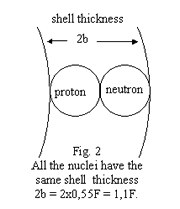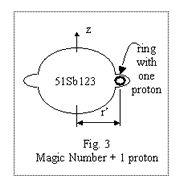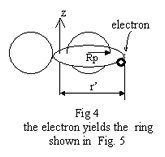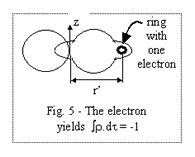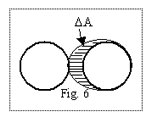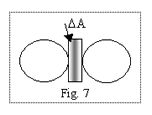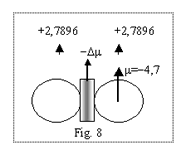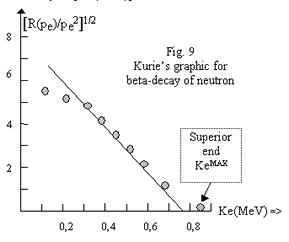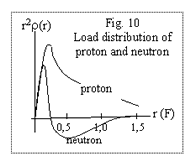### 759 comments to Anomalous mass of the neutron

• Obviously, the world desperately needs an E-Cat powered car. Thus, we will require far higher safe operating temperatures in future E-Cat designs. We need thousands of minds in laboratories all over the world working on this problem. Once people really understand that the technology works, then I think every major energy and transportation related corporation on the planet will do all they can to help. Hopefully, that mass realization will arrive soon. I would hope the Obama Administration would help by speeding the patent process so that full disclosure of the design could be made public.

• raul heining

Dear Rossi,
I have to congratulate you for the way you have, until know, conducted something so
as sensible as this technology and also the way you are always willing to answer us
all the questionos we have ben putting here and your capacity to ignore insults from
people we all know for some months now.
Regards
raul

• Andrea Rossi

Dear Max Altana:
Yes, and also in Uppsala.
Warm Regards,
A.R.

• Andrea Rossi

Dear Dave Price:
Yes, R&D with Bologna and Uppsala Universities is the next scientific step, while the manufacturing and commercialization go on.
Warm Regards,
A.R.

• Andrea Rossi

Dear H. Visscher:
USA, industrial, heating system.
Warm Regards,
A.R.

• Andrea Rossi

Dear Ivan Idso:
Miniaturization is in perspective, but not a priority.
Warm Regards,
A.R.

• Andrea Rossi

Deare Gunnar Lindberg:
It does not depend on me.
Warm Regards,
A.R.

• Andrea Rossi

Dear Russell Varriale:
Expand production: lavolale, lavolale!
Warm Regards,
A.R.

• Andrea Rossi

Dear Robert Mockan:
We do not use radioactive materials and do not leave radioactive waste, while operating do not leave radiations outside the plant, as clearly has been evidenced on the 28th test. All this will help.
Warm Regards,
A.R.

• Andrea Rossi

Dear Robert Mockan:
Interesting consideration.
Warm Regards,
A.R.

• Andrea Rossi

Dear Peter Fimmel:
I agree with you: atomic nucleus is still an unknown world, in great part. CERN is making a very important effort in this sense, though.
Warm Regards,
A.R.

• Andrea Rossi

I made what everybody can make: do my work honestly focusing all my strength in the right point at the right moment. Brain can be used as a lamp or as a laser, depending on the coherence of all the cells dedicated to the work.
Warm Regards,
A.R.

Carissimo Ing. Rossi, adesso ce lo può dire: dove è nascosta la Sua astronave?
Non so come descrivere il nostro stato d’animo… Grazie!

• Peter Fimmel

Congratulations Andrea. You are continuing the tradition of those great Italian scientists from Galilei to Fermi. Your work demonstrates beautifully that the atomic nucleus contains far more secrets than have so far been revealed.

• Robert Mockan

Fascinating data about the demo. The total system weight was 10 tons but that includes a steel shipping container. If optimized for weight no question total weight could be reduced to 1/10 of that, or 1 ton total weight. Rossi had good reasons to go with small modules that could be hooked together, but using standard manufacturing scale factors pertaining to pressure containers the mass could be substantially reduced going to one module putting out a megawatt. So one ton reactor weight per megawatt is reasonable.
Technical problem caused power out to be just under 1/2 megawatt, but the weight analysis still holds for future designs. Now about temperature. Thermodynamics will limit total thermal power to electricity conversion to probably between 2 percent and 4 percent. (There are many reasons for this low efficiency, but the numbers are based on “bottoming” cycles in industry that use waste heat for power generation at comparable temperatures, so are based on practicality, not just theoretical maximum that only seem to indicate greater net efficiency). Let us go with just 3% conversion. 3 percent conversion to electrical of a megawatt thermal would be 30,000 watts. That could drive about a 40 horsepower electric motor. Or use the shaft horsepower directly from the heat engine (steam engine). This would be good for marine applications, since no fuel cost. A power boat could literally circumnavigate the globe, without any problem of logistics for fuel, at low cost for fuel. This is looking interesting. Live aboard sail boats are nice, but now live aboard power boats!
Set up camp on the coast of Antarctica when you have a megawatt nuclear reactor? Why not?

• Robert Mockan

I few obvious kinds of companies would be interested in the E-Cat.
Battelle Corporation, W.C. Grace and Co., ExonMobile, and so on.
These kind of industrial conglomerates could purchase the 1.5 MW E-Cat demo unit what for them is less than pocket change, and they would have full disclosure of every aspect of its development and operation once they purchased it.
It will be interesting to see how this is all going down. Will we see E-Cats in the “near” future, or will “regulations” about the use of “nuclear” technology delay it for many years?

• Russell Varriale

Congratulations! I was wondering, now that you have a paying customer (assuming they have committed finances) what are your next steps? What is your end goal with this?

Thank you.

• Gunnar Lindberg

Dear Andrea Rossi,
My warmest congratulations to the results on October 28. As I am sure you understand, many of your admirers are dissapointed you cannot reveal the name of your first Costumer. We all hope that the next buyer will be more open to the public. Do already you know who it will be?
My best Regards
Gunnar Lindberg

• Ivan Idso

Dear mr. Rossi,

Congradulations on your successful test! You are an amazing engineer, I’m excited to see what you will accomplish in the next year. Pocket watch e-cats?

Sincerely,
Ivan Idso

• H. Visscher

Hello Andrea,

I congratulate you on this commercial and scientific achievement. I have just one question: When will the next delivery take place? What device will that be?

Thanks again for keeping us informed.

H. Visscher

• insight

Dear A.R.
congratulations!!

• Great news, I hope this works out.

Are there any future dates we can look to? I understand there are two university contracts in the works…

• Max Altana

Dear Dr Rossi

I’m happy to hear the 1MW eCat was sold to the secret costumer!

this means that the testing of the eCat in DF of Bologna University will start soon!

please tell us it is so!

Cheers.
M.A.

• Andrea Rossi

Dear Max:
The Customer is of a category that usually maintains secret all they do. I do not know if and when they will want to make public statements and I am bound to a strict non disclosure agreement.
Warm Regards,
A.R.

• Andrea Rossi

Dear Devis,
Of course I will remember always all the friends who have encouraged the efforts of my team and mine.
I thank all with all my heart, and this is dedicated also to all the Friends who have sent all these comments, so heart warming, to which I can’t answer, being again under pressure. A big, big hug to all.
Andrea Rossi

• Max

Will the name of the 1MW E-Cat customer be ever published, and if yes, when?

Congratulations on the successful conclusion of the test!

Max

• Andrea Rossi

Dear James Bowery:
max 20 mm in water column was the pressure at the output of the thermocouple.
The genset has been not turned off because we had to give energy to all the auxiliary motors: water pumps and electric fans of the heat dissipators. Of course the energy consumed from these utilities has not to be put in the energy balance of the reactors, because in an industrial application the energy is not dissipated, is utilized, and the pumps to move the water are necessary in this particular kind of test. In any case, all this is described in the draft report on
http://db.tt/wu4OLbgk
Warm Regards,
A.R.
Warm regards,
A.R.

• What was the pressure at the output thermocouple?
Why the electric power generator (genset) has not been turned off during the self sustained mode?

• Rainer Gruenzfelder

Dear Mr. Rossi,
Congratulations, you did it, the consequences are out of all our imaginations.
You are entitled for the Nobel Price. But let us not forget Mr, Fleischmann who was the first one who raised his head above the establishment. He found the hint, you found the way.
I was working on a similar idea than you, but I was many years behind. You found such an elegant way to bypass the main problem. For this I really admire you.
I am a German, but I am now for 17 years in Chinese environment. I have a very close relationship to the Chinese government based on my GM function in a big German company.
If I can be of any help, please let me know.

I am looking forward to watch you progress and hope in the very near future your invention will become a central part within my living environment.

Congratulations again and all my best wishes

RG

• Mauro Rossi

Historical result!

Andrea may I suggest, after some sleep, to complete the Excel report with Energy and Power calculations,
showing with Specific Heat and Latent Heat formulas that Power is in average around 460 kW when water boils?
[I mean I’ve obtained those results]

The COPS can be higher that 460/66 ratio, because the system goes in self sustaining mode.

It is important also to know how fast the Core can be turned OFF, thinking of a possible Emergency Shut-Down for an House Heating application for the masses. I’m so curious: Is there a pressure valve in the system?

I’m looking forward to all advancements in Physics that your discovery will produce!!!
Thanks to Rossi and thanks to Focardi.

Mauro Rossi

• Mirco Vantaggio

The most sincere congratulations Dr. Andrea Rossi. I followed his work through this BLOG and I rooted for her and for us all. Your name will be remembered in the history.

I wish you a good day
Mirco

• Martin Maurer

Dear Dr. Rossi,

Congratulations, I can’t wait to see how the world will change from now on.
God bless you.

-martin maurer

• oscar galli

Something wonderful is happening, Andrea Rossi invented the “New Clean Energy”.
Oscar Galli

• Artioli Cesarino

Buon Giorno Ing. Rossi

Vorrei farle le congratulazioni per il Test appena svolto, ennesima dimostrazione da parte sua di quando il lavoro e l’ingegno supportati dalla dedizione e dalla volontà possano donare al mondo nuove importantissime scoperte.
Devo ammettere anche che l’emettitore di onde elettromagnetiche ( con funzionalità di stabilizzazione più che di innesco se non ho capito male ) che è stato palesato durante il penultimo test pubblico e quella frase che ha pronunciato in uno spezzone video (riporto rigorosamente in modo non fedele) ” all’interno del reattore scorre corrente elettrica ma …. ” sorridendo facendo intendere che la funzionalità di tale corrente non è per nulla di facile o ovvia intuizione ( presuppongo non si tratti solamente di una banale resistenza… ) hanno acceso in modo preoccupante la mia sete di conoscenza e curiosità.
Trovo che la presunta violazione della velocità dei neutrini tachionici , che si è evidenziata nell’esperimento”Opera”, predetta negli anni trenta da Ettore Majorana e descritta come un effetto del passaggio di queste leggerissime particelle nella materia condensata ponga ancor più l’attenzione su una nuova e eccitante fisica che tenga in debita considerazione le differenze che possono sussistere tra una fisica classica fatta di interazioni in spazi idealmente “vuoti” ad una che ne consideri debitamente gli ambienti complessi molto più realistici.
Spero che al più presto possa essere nelle condizioni di svelare l’impianto teorico sul quale si basa la sua invenzione.
In Attesa che ci sveli il famoso Catalizzatore mi aggiungo idealmente alla schiera di chi prova ad indovinarlo grazie alla propria sensibilità … Io dico il Grafene se non addirittura un campo elettromagnetico calibrato rispetto alla fisica adronica…. Il perché ….. primo perché è estremamente di moda (e poi è stato scoperto nel 2004 anno molto vicino all’inizio delle sue ricerche se non ho capito male ) e secondo sembra che ci si possa fare di tutto …. Non per niente io non sono laureato in fisica ma in economia : )
Grazie della pazienza
Cordiali Salti
Artioli Cesarino

• Carlo M.

To: Andrea Rossi

After long months … thank you so much for your foresight and perseverance!

God bless you.

• Living exciting moments. Complimenti Dr. Rossi, and all your team crew.

• Chris MIlls

Congratulations Dr. Rossi!

• Pierre Duflos

Extraordinaire, félicitations Mr Rossi, ca va changer le monde

• Enzo Amato

Caro Rossi,

adesso vorrei vedere le facce dei suoi detrattori…

Un sincero abbraccio e auguri per il futuro!

Enzo Amato

• Dear Dr. Rossi,

Thank you for involving all of us in your great achievement for the good of mankind.
You have achieved clean, infinite energy production.
“When the input energy is zero, the energy-out over energy-in ratio becomes infinite.”

Best Regards,

Daniel G. Zavela

• Stephen T.

Dear Andrea Rossi,
Congratulations! Thank You for allowing Mats Lewan, Danielle Passarini and Sterling Allen to give us all an inside glimpse of history in the making. It really was wonderful coverage and so appropriately done by you!
Sincerely and Warmly,
Stephen Taylor

• […] Rossi did get one blog post up (edited to convert all-caps to proper-caps and fix an obvious typo): Andrea RossiOctober 28th, 2011 at 10:37 AM […]

• Dear all,
Here’s Ny Teknik’s report on the October 28 test:

Half a megawatt E-cat in Bologna

Kind Regards,
Mats Lewan

• fab70

Congratulations!

• João Corvelo

Parabéns Engº Rossi,
Hoje 28 de Outubro 2011 chegou o Futuro.
Votos de Boa Sorte.
Cumprimentos,

J.Corvelo

• tj

Andrea: Congratulations and Infinite Thanks to All For 470 kWh Infinite COP

• Ron Cisco

Using your averages it appears as though your claims are correct however I am concerned that steam flow rate was not measured. Also for input power it says 66 KWH. Was this total metered power or per hour? How do we know that the heated water was ever turned into steam and that you overcame the heat of vaporization? Thanks.

• Oscar

Una cosa meravigliosa sta accadendo …..
Andrea Rossi ha inventato l’energia pulita.

• […] Rossi did get one blog post up (edited to convert all-caps to proper-caps and fix an obvious typo): Andrea RossiOctober 28th, 2011 at 10:37 AM […]

• Enrica Fiorini

Complimenti a lei e a tutta la sua squadra per la perseveranza e il coraggio che avete dimostrato lavorando su idee innovative in opposizione alle posizioni della maggior parte del mondo accademico. E’ molto difficile mantenere la propria direzione viaggiando contro corrente, dovendo sopportare continue difficoltà, opposizioni e derisioni da parte di molti. Spero per lei in un futuro di pieno riscatto.
Grazie per questa bella favola che speriamo sia veramente happy ending per l’umanità.